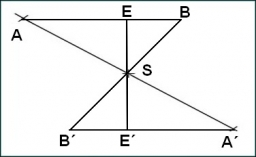# Circumference 9811

Kristýna chose a certain odd natural number divisible by three. Jakub and David then examined triangles with a circumference in millimeters equal to the number selected by Kristýna and whose sides have lengths in millimeters expressed by different integers.
Jakub found a triangle in which the longest side had the longest possible length and wrote this value on the board. David found a triangle in which the shortest side has the longest possible length and wrote this value on the board. Kristýna correctly added both measurements on the board and got 1,681 mm.
Determine which number Kristýna chose.

K =  2019

### Step-by-step explanation:Did you find an error or inaccuracy? Feel free to write us. Thank you!

Tips for related online calculators
Do you have a linear equation or system of equations and looking for its solution? Or do you have a quadratic equation?
Do you solve Diofant problems and looking for a calculator of Diofant integer equations?
Do you want to round the number?